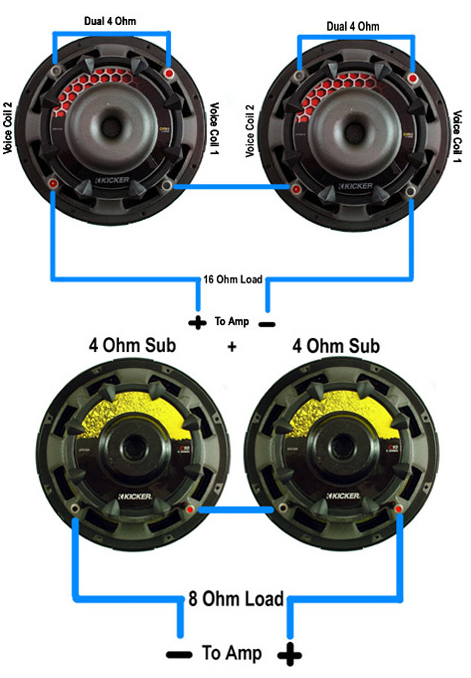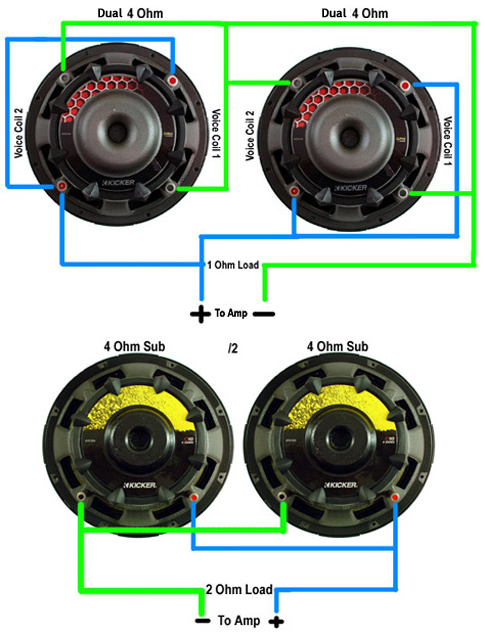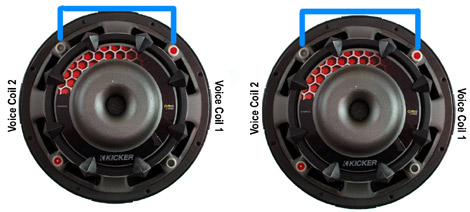# Wiring Subwoofers & Speakers To Change Ohm’s

The ohm is a measurement of the electric resistance between two points. In a speaker, the ohm is a bad thing. The lower the ohms the less energy it takes to drive the speaker. The less energy, the more you get out of your amps. An 8 ohm speaker requires more amperage to run than a 4 ohm speaker. You need to know the ohms of all of your speakers if you want to calculate the load your amp is taking and to compensate for it.

In a car audio you should always run your equipment at the lowest possible Ohm load that an amplifier, head unit or speaker can safely handle. All amplifiers will feature a rating which will usually look something like this.

• 500 Watts x 1 @ 4 Ohm
• 1000 Watts x 1 @ 2 Ohm

Now this is really important when purchasing equipment. To get the maximum output out of the amplifier rating listed above you want to make sure it is wired at a 2 Ohm load, running the same amplifier at a 4 Ohm load will result in a 50% loss in potential power. The same applies the other way around. You would not want to run this amplifier lower than a 2 Ohm load otherwise you can potentially burn it up since it would be running at twice the recommended power rating and capabilities. Most amplifiers have a thermal protection circuit however, it is still possible to damage the amplifier. Now if you look at my diagram above you can really see how it works. The larger pipe above would represent a 2 Ohm load whereas the smaller pipe would be 4 Ohm. Every time an Ohm load is divided by 2 the current will double in amperage. Every time an Ohm load is multiplied by 2 the current will be cut by half.

Next we will look at subwoofers and how to wire them properly to the amplifier. Using the same amplifier ratings from above we now know that we want to wire the amp at a 2 Ohm load. So how do we do that? This is where things tend to get a little trickier however it is not as hard as it may seem. There are two terms that you will need to know when wiring subs or speakers, Parallel and Series. Lets look at each example before we figure out exactly how to wire our amplifier example from above.  Just remember wiring in series it is used to INCREASE the Ohm load and parallel is used to DECREASE the Ohm load. So an example of a series circuit can be viewed below.When wiring in series you are increasing the Ohm load by hopping from one voice coil to the next. So as you can see pictured the positive hops to negative on the opposite voice coil. To add this up you take the 4 Ohm load of each coil and add them together. So if you are wiring two Single 4 Ohm subs together you would add the two voice coils together sp 4 Ohm + 4 Ohm equals an end result of 8 Ohm. Moving on, an example of a parallel circuit can be seen below:When wiring in parallel you are decreasing the Ohm load and wiring the voice coils together.  So you would run a lead from the positive voice coil on each sub to the opposite voice coil. In essence all the positives are connected on a single wire (conductor) and the same for the negatives. So if wiring two Single 4 Ohm subs you would take 4 Ohm and divide it by the number of voice coils. So 4 Ohm divided by two voice coils will equal a 2 Ohm load.

Okay now as you saw above the single 4 Ohm subs wired in a parallel resulted in a 2 Ohm load, just what we needed for the amplifier example from above. But the dual 2 Ohm subwoofers came to a 1 ohm load that’s too low, what do we do? You will normally have to use a combination of series and parallel to achieve the correct Ohm load. Let’s say you are running the amplifier I mentioned above. That amplifier will need to run at a 2 Ohm load to achieve maximum power. So if you decide you wanted to run two subwoofers on that amplifier you would need to run either two single 4 Ohm subwoofers or two dual 2 Ohm subwoofers. The two single 4 Ohm subs are easy to wire and you can see that in the diagram above. However, the dual 2 Ohm subs will use a combination: first we wire each subwoofer in a series and then wire them together in parallel at the amplifier. How do we do that? Lets go over this in a diagram. Think of it as a two step process this will make it easier to figure out. So first you would take the subs and wire them in series like below.Ok so that is first step. So now you have two Dual 2 Ohm subs wired in series. This increases the Ohm load to 4 Ohm at each sub. Now we need to wire them in parallel at the amplifier. Check out the diagram below.As you can see we now used a combination of first wiring each sub in series, and then we wired the subwoofers in parallel at the amplifier. This would be a typical installation and can be found in many installs. These are just a few examples but once you have the basics down you can figure out much more complex systems using the same methods.